# Close-packing of spheres

Close-packing of spheres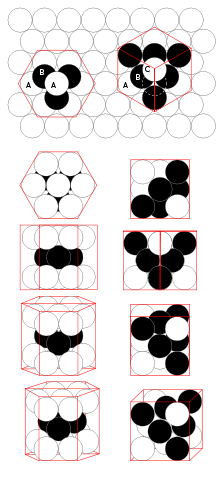hcp and fcc close-packing of spheres

In geometry, close-packing of spheres is a dense arrangement of equal spheres in an infinite, regular arrangement (or lattice). Carl Friedrich Gauss proved that the highest average density – that is, the greatest fraction of space occupied by spheres – that can be achieved by a regular lattice arrangement is$\frac{\pi}{3\sqrt 2} \simeq 0.74048.$

The Kepler conjecture states that this is the highest density that can be achieved by any arrangement of spheres, either regular or irregular. This conjecture is now widely considered proven by T. C. Hales.

Many crystal structures are based on a close-packing of atoms, or of large ions with smaller ions filling the spaces between them. The cubic and hexagonal arrangements are very close to one another in energy, and it may be difficult to predict which form will be preferred from first principles.

## fcc and hcp lattices

There are two simple regular lattices that achieve this highest average density. They are called face-centered cubic (fcc) (also called cubic close packed) and hexagonal close-packed (hcp), based on their symmetry. Both are based upon sheets of spheres arranged at the vertices of a triangular tiling; they differ in how the sheets are stacked upon one another.

### Cannonball problem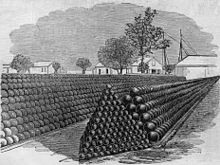Cannonballs piled on a triangular (front) and rectangular (back) base, both fcc lattices.

The problem of close-packing of spheres was first mathematically analyzed by Thomas Harriot around 1587, after a question on piling cannonballs on ships was posed to him by Sir Walter Raleigh on their expedition to America. Cannonballs were usually piled in a rectangular or triangular wooden frame, forming a three-sided or four-sided pyramid. Both arrangements produce a face-centered cubic lattice – with different orientation to the ground.

### Positioning and spacing

In both the fcc and hcp arrangements each sphere has twelve neighbors. For every sphere there is one gap surrounded by six spheres (octahedral) and two smaller gaps surrounded by four spheres (tetrahedral). The distances to the centers of these gaps from the centers of the surrounding spheres is$\sqrt{\frac{3}{2}}$ for the tetrahedral, and$\sqrt2$ for the octahedral, when the sphere radius is 1.

Relative to a reference layer with positioning A, two more positionings B and C are possible. Every sequence of A, B, and C without immediate repetition of the same one is possible and gives an equally dense packing for spheres of a given radius.

The most regular ones are:

• fcc = ABCABCA (every third layer is the same)
• hcp = ABABABA (every other layer is the same)

In close-packing, the center-to-center spacing of spheres in the xy plane is a simple honeycomb-like tessellation with a pitch (distance between sphere centers) of one sphere diameter. The distance between sphere centers, projected on the z (vertical) axis, is:$\text{pitch}_Z = \sqrt{6} \cdot {d\over 3}\approx0.81649658 d,$

where d is the diameter of a sphere; this follows from the tetrahedral arrangement of close-packed spheres.

The coordination number of hcp and fcc is 12 and its atomic packing factor (APF) is the number mentioned above, 0.74.

Comparison between hcp and fcc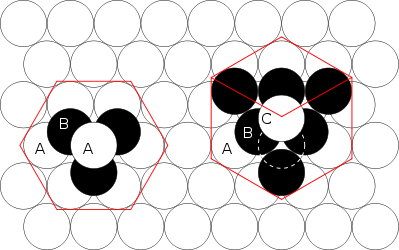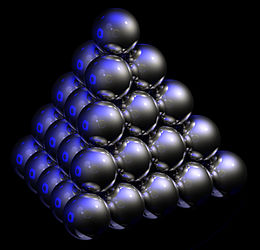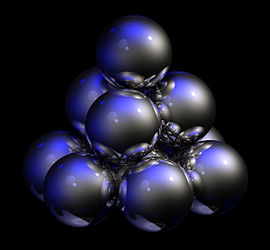Figure 1 – The hcp lattice (left) and the fcc lattice (right). The outline of each respective Bravais lattice is shown in red. The letters indicate which layers are the same. There are two "A" layers in the hcp matrix, where all the spheres are in the same position. All three layers in the fcc stack are different. Note the fcc stacking may be converted to the hcp stacking by translation of the upper-most sphere, as shown by the dashed outline. Figure 2Thomas Harriot, circa 1585, first pondered the mathematics of the cannonball arrangement or cannonball stack, which has an fcc lattice. Note how adjacent balls along each edge of the regular tetrahedron enclosing the stack are all in direct contact with one another. This does not occur in an hcp lattice, as shown in Fig. 3. Figure 3 – Shown here is a stack of eleven spheres of the hcp lattice illustrated in Fig. 1. The hcp stack differs from the top 3 tiers of the fcc stack shown in Fig. 2 only in the lowest tier; it can be modified to fcc by an appropriate rotation or translation.

## Lattice generation

When forming any sphere-packing lattice, the first fact to notice is that whenever two spheres touch a straight line may be drawn from the center of one sphere to the center of the other intersecting the point of contact. The distance between the centers along the shortest path namely that straight line will therefore be r1 + r2 where r1 is the radius of the first sphere and r2 is the radius of the second. In close packing all of the spheres share a common radius, r. Therefore two centers would simply have a distance 2r.

### Simple hcp latticeAn animation of hcp lattice generation.

To form an A-B-A-B-... hexagonal close packing of spheres, the coordinate points of the lattice will be the spheres' centers. Suppose, the goal is to fill a box with spheres according to hcp. The box would be placed on the x-y-z coordinate space.

First form a row of spheres. The centers will all lie on a straight line. Their x-coordinate will vary by 2r since the distance between each center if the spheres are touching is 2r. The y-coordinate and z-coordinate will be the same. For simplicity, say that the balls are the first row and that their y- and z-coordinates are simply r, so that their surfaces rest on the zero-planes. Coordinates of the centers of the first row will look like (2rrr), (4rrr), (6r ,rr), (8r ,rr), ... . The sphere centered at x = 0 is immediately omitted because part of the sphere would lie outside.

Now, form the next row of spheres. Again, the centers will all lie on a straight line with x-coordinate differences of 2r, but there will be a shift of distance r in the x-direction so that the center of every sphere in this row aligns with the x-coordinate of where two spheres touch in the first row. This allows the spheres of the new row to slide in closer to the first row until all spheres in the new row are touching two spheres of the first row. Since the new spheres touch two spheres, their centers form an equilateral triangle with those two neighbors' centers. The side lengths are all 2r, so the height or y-coordinate difference between the rows is$\scriptstyle\sqrt{3}r$. Thus, this row will have coordinates like this:$(r, r + \sqrt{3}r, r),\ (3r, r + \sqrt{3}r, r),\ (5r, r + \sqrt{3}r, r),\ (7r, r + \sqrt{3}r, r), \dots$

The first sphere of this row only touches one sphere in the original row, but its location follows suit with the rest of the row.

The next row follows this pattern of shifting the x-coordinate by r and the y-coordinate by$\scriptstyle\sqrt{3}$. Add rows until reaching the x and y maximum borders of the box.

In an A-B-A-B-... stacking pattern, the odd numbered planes of spheres will have exactly the same coordinates save for a pitch difference in the z-coordinates and the even numbered planes of spheres will share the same x- and y-coordinates. Both types of planes are formed using the pattern mentioned above, but the starting place for the first row's first sphere will be different.

Using the plane described precisely above as plane #1, the A plane, place a sphere on top of this plane so that it lies touching three spheres in the A-plane. The three spheres are all already touching each other, forming an equilateral triangle, and since they all touch the new sphere, the four centers form a regular tetrahedron. All of the sides are equal to 2r because all of the sides are formed by two spheres touching. The height of which or the z-coordinate difference between the two "planes" is$\scriptstyle\sqrt{6}r2/3$. This, combined with the offsets in the x and y-coordinates gives the centers of the first row in the B plane:$(r,\sqrt{3}r/3,r+\sqrt{6}r2/3),\ (3r,\sqrt{3}r/3,r+\sqrt{6}r2/3),\ (5r,\sqrt{3}r/3,r+\sqrt{6}r2/3),\ (7r,\sqrt{3}r/3,r+\sqrt{6}r2/3),\dots$

The second row's coordinates follow the pattern first described above and are:$(2r,4\sqrt{3}r/3,r+\sqrt{6}r2/3),\ (4r, 4\sqrt{3}r/3, r + \sqrt{6}r2/3),\ (6r,4\sqrt{3}r/3, r + \sqrt{6}r2/3),\ (8r, 4\sqrt{3}r/3,r + \sqrt{6}r2/3),\dots$

The difference to the next plane, the A plane, is again$\sqrt{6}r2/3$ in the z-direction and a shift in the x and y to match those x- and y-coordinates of the first A plane.

## Miller indices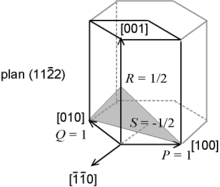Miller–Bravais index for hcp lattice

Crystallographic features of hcp systems, such as vectors and atomic plane families can be described using a four-value Miller index notation ( hkil ) in which the third index i denotes a convenient but degenerate component which is equal to −h − k. The h, i and k index directions are separated by 120°, and are thus not orthogonal; the l component is mutually perpendicular to the h, i and k index directions.

Wikimedia Foundation. 2010.

Нужен реферат?

### Look at other dictionaries:

• Sphere packing — In mathematics, sphere packing problems are problems concerning arrangements of non overlapping identical spheres which fill a space. Usually the space involved is three dimensional Euclidean space. However, sphere packing problems can be… …   Wikipedia

• Random close pack — Random close packing (RCP) is an empirical parameter used to characterize the maximum volume fraction of solid objects obtained when they are packed randomly. For example, when a solid container is filled with grain, shaking the container will… …   Wikipedia

• Atomic packing factor — In crystallography, atomic packing factor (APF) or packing fraction is the fraction of volume in a crystal structure that is occupied by atoms. It is dimensionless and always less than unity. For practical purposes, the APF of a crystal structure …   Wikipedia

• Molecular model — A molecular model, in this article, is a physical model that represents molecules and their processes. The creation of mathematical models of molecular properties and behaviour is molecular modelling, and their graphical depiction is molecular… …   Wikipedia

• Buckminster Fuller — Infobox Person name = R. Buckminster Fuller image size = 200px caption = R. Buckminster Fuller c.1917 birth date = birth date|1895|07|12 birth place = Milton, Massachusetts death date = death date and age|1983|7|1|1895|07|12|mf=y death place =… …   Wikipedia

• silica mineral — Any of the forms of silicon dioxide (SiO2), including quartz, tridymite, cristobalite, coesite, stishovite, melanophlogite, lechatelierite, and chalcedony. Various kinds of silica minerals have been produced synthetically. * * * Introduction… …   Universalium

• Phyllotaxis — Crisscrossing spirals found in nature In botany, phyllotaxis or phyllotaxy is the arrangement of leaves on a plant stem (from Ancient Greek phýllon leaf and táxis arrangement ). …   Wikipedia

• List of mathematics articles (C) — NOTOC C C closed subgroup C minimal theory C normal subgroup C number C semiring C space C symmetry C* algebra C0 semigroup CA group Cabal (set theory) Cabibbo Kobayashi Maskawa matrix Cabinet projection Cable knot Cabri Geometry Cabtaxi number… …   Wikipedia

• Convex uniform honeycomb — The alternated cubic honeycomb is one of 28 space filling uniform tessellations in Euclidean 3 space, composed of alternating yellow tetrahedra and red octahedra. In geometry, a convex uniform honeycomb is a uniform tessellation which fills three …   Wikipedia

• Waterman butterfly projection — The Waterman Butterfly World Map Projection was created by Steve Waterman and published in 1996. It is an octahedral transformation of a globe, reviving the Butterfly Map principle first developed by Bernard J.S. Cahill (1866 1944) in 1909.… …   Wikipedia